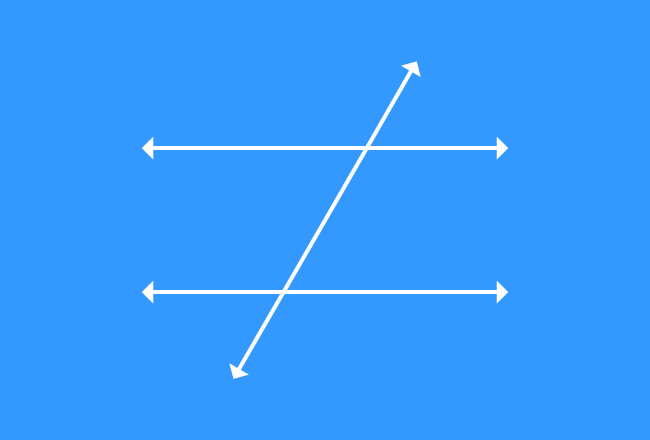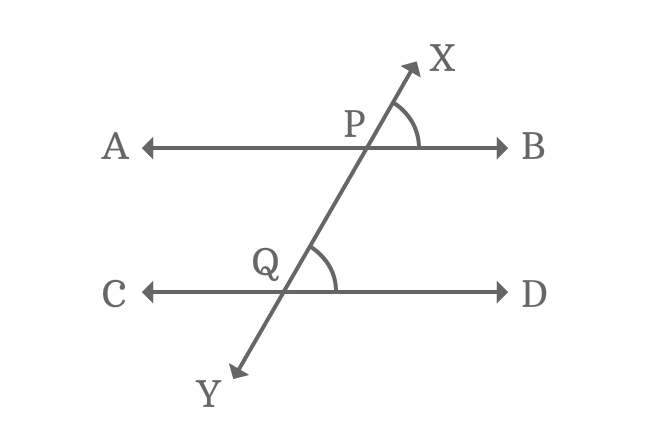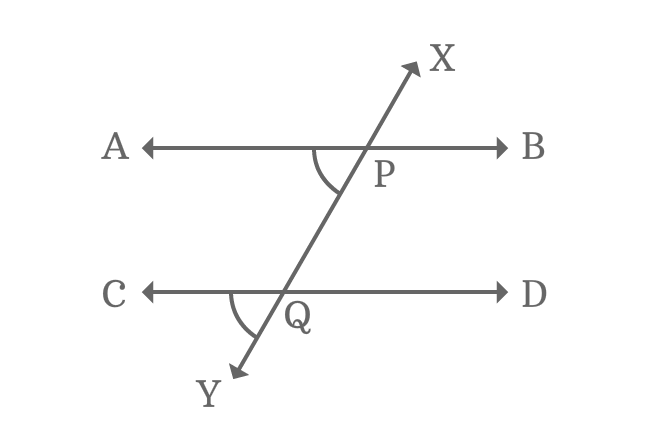# Corresponding Angles formed by Parallel Lines and their transversalIn the case of intersection of two parallel lines by their transversal, four pairs of angles have same relative position at each intersection of every two straight lines and they are known as corresponding angles of the transversal of parallel lines.

Look at the picture, an angle has similarity in position with another angle. Each pair of such angles are corresponding angles and total four pairs of corresponding angles are possibly formed geometrically when a pair of parallel lines are intersected by their transversal line.

## Property

The corresponding angles which have relative position are equal when two parallel lines are cut by their transversal and the property can be proved geometrically.$\angle XPB$ and $\angle XQD$ are two angles and they are relatively similar. Therefore, they both are called as corresponding angles. The two corresponding angles are equal geometrically when two parallel lines $\overleftrightarrow{AB}$ and $\overleftrightarrow{CD}$ are intersected by their transversal $\overleftrightarrow{XY}$.

$\angle XPB = \angle XQD$

Similarly, the corresponding angles $\angle YPB$ and $\angle YQD$ are also equal geometrically.

$\angle YPB = \angle YQD$The corresponding angles $\angle YQC$ and $\angle YPA$ are equal because of similarity in position.

$\angle YQC = \angle YPA$

Finally, the pair of corresponding angles $\angle XQC$ and $\angle XPA$ are also equal.

$\angle XQC = \angle XPA$

Therefore, the animation tutorial has proved that each pair of corresponding angles are equal geometrically when two parallel lines are cut by their transversal line.

Latest Math Topics
Jun 26, 2023
Jun 23, 2023

Latest Math Problems
Jul 01, 2023
Jun 25, 2023
###### Math Questions

The math problems with solutions to learn how to solve a problem.

Learn solutions

Practice now

###### Math Videos

The math videos tutorials with visual graphics to learn every concept.

Watch now

###### Subscribe us

Get the latest math updates from the Math Doubts by subscribing us.